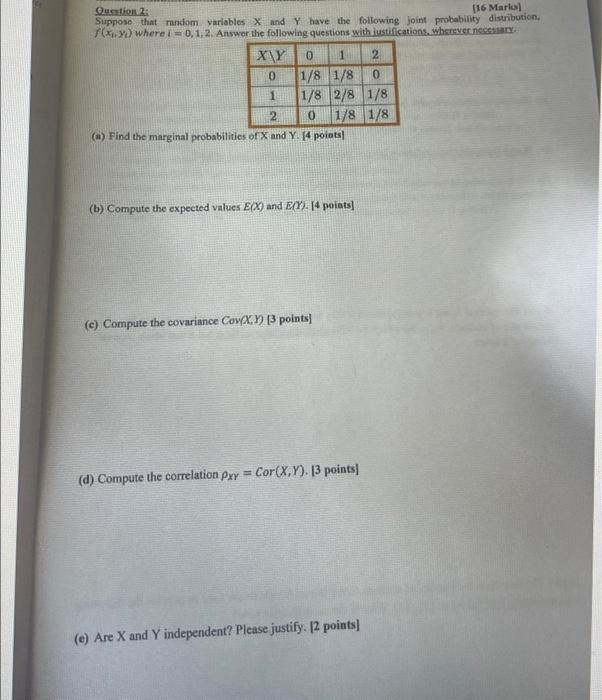Home / Expert Answers / Statistics and Probability / question-2-16-marks-suppose-that-random-variables-x-and-y-have-the-following-joint-probability-di-pa421

# (Solved): Question 2: [16 Marks] Suppose that random variables X and Y have the following joint probability di ...

Question 2: [16 Marks] Suppose that random variables X and Y have the following joint probability distribution, f(x,y) where i = 0, 1, 2. Answer the following questions with justifications, wherever necessary. 1 2 1/8 1/8 0 1/8 2/8 1/8 0 1/8 1/8 0 1 2 (a) Find the marginal probabilities of X and Y. [4 points] (b) Compute the expected values E(X) and E(Y). [4 points] (c) Compute the covariance Cov(X,Y) [3 points] (d) Compute the correlation pxy Cor(X,Y). [3 points] = (e) Are X and Y independent? Please justify. [2 points]Quntion 2: [16 Marks] Supposo that mandom variables and have the following joint probability distribution. where . Answer the following questions with iustifications, wherevecincesturd. (a) Find the marginal probabilities of and . [4 points] (b) Compute the expected values and . [4 poiats] (c) Compute the covariance points] (d) Compute the correlation points (e) Are and independent? Please justify. [2 points]

We have an Answer from Expert